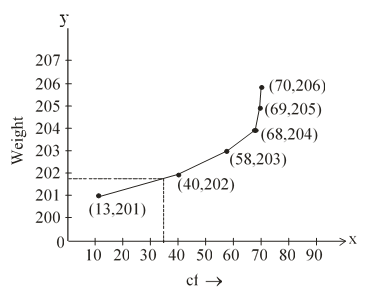#### The weights of tea in 70 packets are shown in the following table :   Weight (in gram) Number of packets 200-201 201-202 202-203 203-204 204-205 205-206 13 27 18 10 1 1 Draw the less than type ogive for this data and use it to find the median weight.Solution.

 Weight cf Less than 201 13 Less than 202 27+13=40 Less than 203 40+18=58 Less than 204 58+10=68 Less than 205 68+1 =69 Less than 206 69+1 = 70Hence the median is 201.8